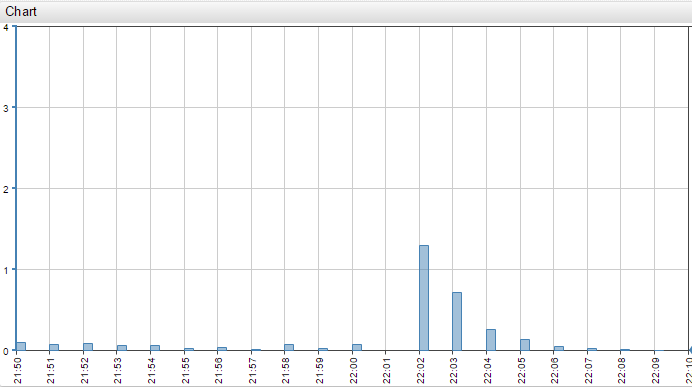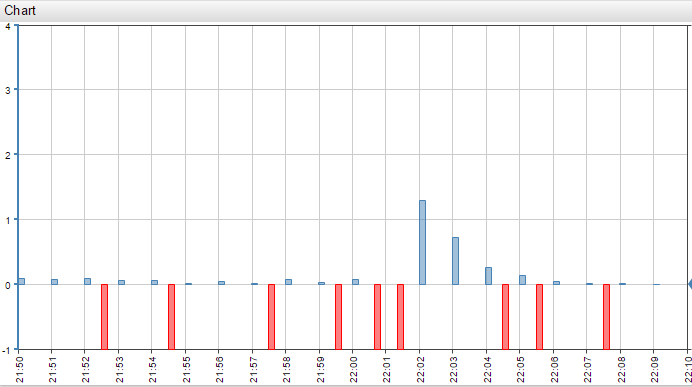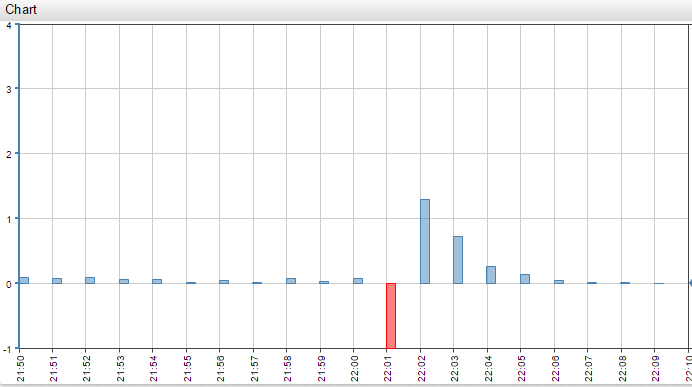# Disconnect Count Behavior

In this example too many missing columns are inserted when using the `disconnect-count = 1` setting. We go over the reasons behind this and how to solve this issue correctly.

Chart Lab: ViewIf `disconnect-count = 1`, then the disconnect interval will be exactly equal to the mean interval.

The problem in this example is that the time interval lengths between adjacent points are all different from one another.

This means that half of them are greater than the mean interval and, as consequently, greater than the disconnect interval.

That’s why we see disconnect values after every other data point.Using `disconnect-count = 1.1`, for example, prevents this problem: